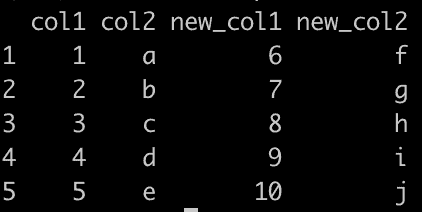# How to Add Column to Data Frame in R

There are four methods to add a column to a data frame in R.

1. Using the “\$” operator
2. Using the “square ([ ])” notation
3. Using the “cbind()” function
4. Using the add_column()” function from the “tidyverse” package

## Method 1: Using the \$ operator

To add a new column in the R data frame, you can use the “\$ operator” and assign a vector to the new column, adding a new column with five rows in the data frame.

### Example

``````mando <- data.frame(
character_id = c(1:5),
character_name = c("Mandalorian", "Grogu", "Ahsoka",
"Bo Katan", "Moff Gideon"),
character_age = c(30, 50, 29, 28, 50),
stringsAsFactors = FALSE
)
# Print the data frame.
mando
rv_new <- c(900, 1000, 600, 400, 800)

mando\$character_bounty <- rv_new

cat("After adding a new column", "\n")
mando
``````

OutputYou can see from the output that the new column character_bounty is added with five values.

## Method 2: Using the Square Brackets

“Square brackets” are another approach to adding a new column in the R data frame.

``mando["character_bounty"] <- rv_new``

Instead of using the \$ operator to attach a new column to the data frame, use the square brackets to attach a new column and assign the values to that column.

### Example

``````mando <- data.frame(
character_id = c(1:5),
character_name = c("Mandalorian", "Grogu", "Ahsoka",
"Bo Katan", "Moff Gideon"),
character_age = c(30, 50, 29, 28, 50),
stringsAsFactors = FALSE
)
# Print the data frame.
mando
rv_new <- c(900, 1000, 600, 400, 800)

# add a new column using [ ] brackets
mando["character_bounty"] <- rv_new
cat("After adding a new column", "\n")
mando
``````

Output## Method 3: Adding a column with the cbind() function in the data frame

The “cbind() function” combines a sequence of vector, matrix, or data frame arguments with columns or rows. That is why the cbind() function can add a column to a data frame as follows.

``mando <- cbind(mando, character_bounty = rv_new)``

The cbind() function takes the data frame, the new column name, and its values. In our case, the new column name is character_bounty, and its value is vector rv_new.

### Example

``````mando <- data.frame(
character_id = c(1:5),
character_name = c("Mandalorian", "Grogu", "Ahsoka",
"Bo Katan", "Moff Gideon"),
character_age = c(30, 50, 29, 28, 50),
stringsAsFactors = FALSE
)
# Print the data frame.
mando
rv_new <- c(900, 1000, 600, 400, 800)

# add a new column using cbind() function
mando <- cbind(mando, character_bounty = rv_new)
cat("After adding a new column", "\n")
mando``````

It will give you the same output as above. As you can see, we have seen three techniques to add a data frame column in r.

### Add Multiple Columns to DataFrame

You can use the “cbind()” function to add multiple columns to a data frame.

``````# Create a data frame
df <- data.frame(
col1 = c(1, 2, 3, 4, 5),
col2 = c("a", "b", "c", "d", "e")
)

# Create new columns
new_col1 <- c(6, 7, 8, 9, 10)
new_col2 <- c("f", "g", "h", "i", "j")

# Add new columns to the data frame
df <- cbind(df, new_col1, new_col2)

# Print the modified data frame
print(df)``````

Output## Method 4: Use the add_column() function from tidyverse

You can use the “add_column()” function from “tidyverse” to add a column to the DataFrame in R.

### Example

``````library(tidyverse)

# Create a data frame
df <- data.frame(
col1 = c(1, 2, 3, 4, 5),
col2 = c("a", "b", "c", "d", "e")
)

# Add new columns to the data frame
df <- add_column(df, new_col = c(11, 12, 13, 14, 15), .after = "col1")

# Print the modified data frame
print(df)
``````

Output

``````  col1  new_col   col2
1  1      11       a
2  2      12       b
3  3      13       c
4  4      14       d
5  5      15       e
``````

That’s it.